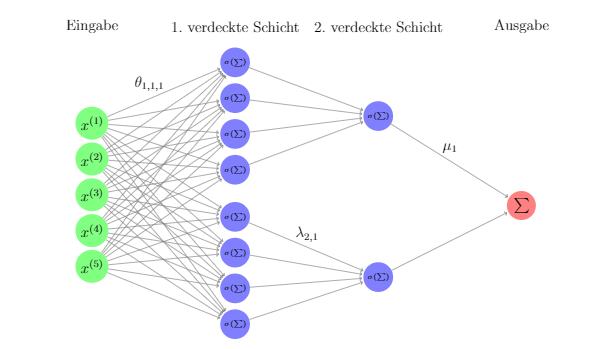# Sparse network doesnt improve

Hi there,

I want to implement a class of sparse neural networks, the picture is an example for the class H_0 for the parameters d = 5, d_star = 1, M_star = 2.

This network class works kind of good, but when I use a random uniform distributed values as input, I get always an almost constant output (untrained).

When I train the network, it gets better sometimes (if I use y_train = f(x_train) as labels for some nonlinear function f).

Unfortunally I want to use this network class, to implement an even deeper network class which uses H_0 networks as its layers.
Because the H_0 give almost constant output, the output of my “bigger” class is completely constant even after training.
Any ideas why the networks behave like that?This is my class, which uses the class smallDense to obtain the sparsity.

```````
import math
import numpy as np

import torch
import torch.nn as nn
import torch.nn.functional as F

class smallDense(nn.Module):
def __init__(self, d, d_star):
super(smallDense, self).__init__()
self.fc1 = nn.Linear(d,4*d_star)
self.fc2 = nn.Linear(4*d_star,1)

def forward(self, input):
x = torch.sigmoid(self.fc1(input))
x = torch.sigmoid(self.fc2(x))
return x

class H_0(nn.Module):
def __init__(self, d, d_star, M_star):
super(H_0, self).__init__()
self.networks = nn.ModuleList([smallDense(d, d_star) for i in range(M_star)])

self.fc_out = nn.Linear(M_star, 1)

def forward(self, input):
outputs = []
for i in range(len(self.networks)):
x = torch.sigmoid(self.networks[i](input))
outputs.append(x)

result = torch.cat(outputs, dim=1)

x = self.fc_out(result)
return x

net = H_0(5,1,3)

x_train = torch.rand(100,5)

net(x_train)`
``````

In `H_0` you are applying `torch.sigmoid` on `self.networks[i](input)`, which has already applied a `sigmoid` on the output, so you are squashing the output again, which might be unwanted.

Besides that I can’t find any obvious issues, but can neither comment if this type of cell would work, so let us know how your experiments worked out.1 Like

Oh, thanks @ptrblck! This was unwanted, but unfortunally it doesn’t really behave differently after changing that.

What I don’t understand is, that the network gives me always outputs close to each other, even though the input differs (alot).

For example this input:

``````net1 = H_0(7,3,2)
net2 = H_0(7,3,2)

x_train = torch.tensor([[-500.0,-600.0,-340,0,-900000,-200,-66]])
x_train1 = torch.ones(1,7)
x_train2 = torch.rand(1,7)

print(net1(x_train))
print(net1(x_train1))
print(net1(x_train2))

print(net2(x_train))
print(net2(x_train1))
print(net2(x_train2))
``````

results in the following output:

``````tensor([[-0.0762858838]], grad_fn=<AddmmBackward>)
``````

For each network the output is kind of constant while the input differs.
I know, that the sigmoid squashes into the interval [0,1] but shouldn’t it differ a bit more?

And if I use the H_0 networks in my bigger network recursively I get outputs that converge to a constant because each H_0 behaves like that.

By the way this network is motivated by a theoretical result for rates of convergence in nonparametric regression using feedforward neural networks by Kohler and Bauer (2019)
Bauer, Benedikt; Kohler, Michael. On deep learning as a remedy for the curse of dimensionality in nonparametric regression.
Ann. Statist. 47 (2019), no. 4, 2261–2285. doi:10.1214/18-AOS1747. https://projecteuclid.org/euclid.aos/1558425645

Not necessarily and you could check all intermediates to have a look what is “happening” in the model:

``````class smallDense(nn.Module):
def __init__(self, d, d_star):
super(smallDense, self).__init__()
self.fc1 = nn.Linear(d,4*d_star)
self.fc2 = nn.Linear(4*d_star,1)

def forward(self, input):
x = torch.sigmoid(self.fc1(input))
print('sigmoid(smallDense.fc1(x)) ', x)
x = torch.sigmoid(self.fc2(x))
print('sigmoid(smallDense.fc2(x)) ', x)
return x

net1 = H_0(7,3,2)
net2 = H_0(7,3,2)

x_train = torch.tensor([[-500.0,-600.0,-340,0,-900000,-200,-66]])
x_train1 = torch.ones(1,7)
x_train2 = torch.rand(1,7)

print(net1(x_train))

print(net1(x_train1))
print(net1(x_train2))

print(net2(x_train))
print(net2(x_train1))
print(net2(x_train2))
``````

You can see that the first input of course saturates the output of `sigmoid(fc1)`. However, since `sigmoid` squashes it to `[0, 1]`, `sigmoid(fc2)` could have “similar” outputs for `x_train` as for `x_train1` and `x_train2` (in my run `tensor([[0.5298]]` vs. `tensor([[0.5521]]`).

That doesn’t mean that the model is not trainable and your model might output larger differences for smaller input changes after training.

1 Like

Okay, that helped alotthank you! Training progress also works fine now on the bigger model!